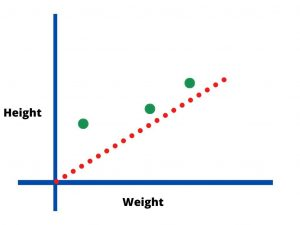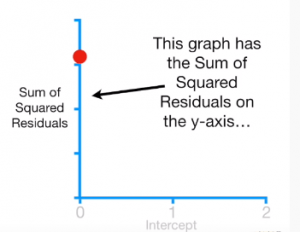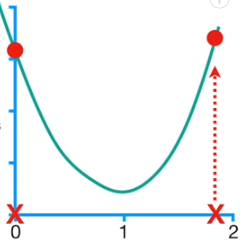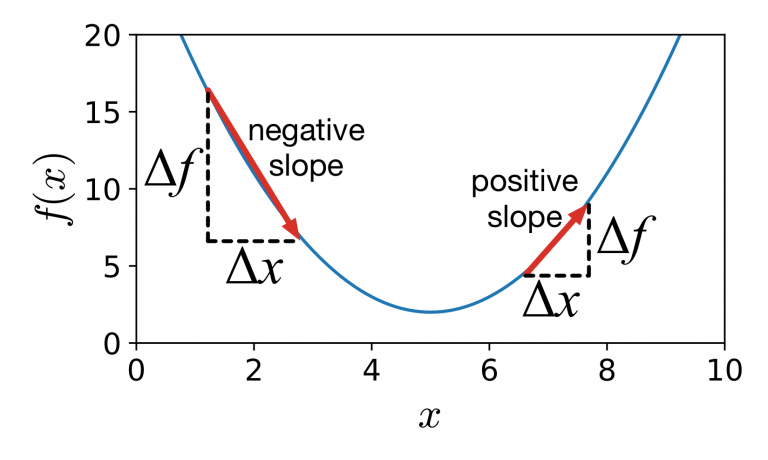# Gradient Descent for Machine Learning

By Learnbay Category Data Science Reading time 5-7 mins Published on Mar 6, 2022

Learn how Gradient Descent is used to train Machine Learning Models

Gradient descent in machine learning refers to a repetitive optimization approach extensively used in training deep learning and machine learning algorithms. It aids in determining a function's local minimum.

Gradient Descent is a popular optimization approach for training ML models by reducing errors across predicted and actual outcomes. In addition, gradient descent neural network has also been in utilization.

## How does Gradient Descent function?

It is an optimization algorithm to find the values of parameters(coefficient) of a function(f) that minimizes the cost function. It is used when parameters can not be calculated by Linear algebra. To minimize the cost function J(w) parameterized by a model parameter w, it helps by telling us about the slope of the cost function. To minimize the cost function, we move toward Gradient descent, which helps by scaling the large dataset.

We can now fit the line with linear regression, a straight line, and squiggle with Logistic regression; we can fit the data into the line with the Gradient descent in machine learning. Gradient descent enhances these and numerous other factors.

For example, we have a simple dataset:

We have weight in X-axis and Height in Y-axis with values (x1,y1)=(0.4, 1.3)

x2,y2= (1.2, 1.6)

x3,y3= (2, 3.1)

We improve the intercept & slope while fitting a line via linear regression.

Height = intercpet+slope*Weight (simple line equation)

Here we can initialize the slope as 0.64 to find the intercept, so plug in the Least square estimate for the slope 0.64 and intercept as 0.Height = intercpet+slope*Weight

Height = intercept+0.64*weight

The first step is to pick a random value for the intercept. This is an initial guess that gradient descent is something to improve upon.

Predicted Height = 0+0.64*0.4

Predicted Height = 0.25

Residual = Observed – Predicted

1.4-0.25 = (1.15)

Similarly, we will calculate the residuals for all three points in the dataset, so all three predicted values are 1.15, 0.84, 1.82

Now we will calculate Sum of Squared Residuals = (1.15)^2+(0.84)^2+(1.82)^2 = 5.34

This is the new value for the y-axis. If we want to plot the graph with a value of 5.34 on the y-axis, we haveThis graph depicts the sum of squared residuals with zero intercept. If we have intercept 0.5, the Sum of squared residuals comes down on the graph. We can find the Sum of squared residuals by changing the value of the intercept and residuals. Gradient descent in machine learning does only a few calculations to find the optimal values and increases the number of calculations closer to the optimal values.

It identifies the optimal values with big steps if they are far from each other and takes baby steps when values are close to each other.After getting all values of the Sum of squared residuals now, we have an equation of a curve. Thus we can take a derivative of this function & determine the slope & value of the intercept.

d/d intercept = d/d intercept*(1.3 – (intercept+ 0.64*0.4))^2

• d/d intercept*(1.6 – (intercept+ 0.64*0.1.2)^2
• d/d intercept*(3.1 – (intercept+ 0.64*2))^2

we will apply the chain rule to solve the derivative

d/d intercept = 2(1.4-(intercept+0.64_0.3))_(-1) so that we have

d/d intercept = -2(1.4-(intercept+0.64*0.3))

• -2(1.6 – (intercept+ 0.64*1.2)
• -2(3.1 – (intercept+ 0.64*2)

Now we have the derivative, so with the help of this derivative, we can find where the Sum of squared residuals is lowest. We will discover the value where the slope value is 0 using the least square approach to obtain the best number of intercepts. Still, with the help of Gradient descent in machine learning, we can find the minimum value by taking steps from the initial guess until we reach the best value.

This makes the GD very efficient when it is impossible to solve for where the derivative is 0. The slope of the curve approaches zero as we get closer to the ideal value of the intercept.

When we are close to the optimal value where the slope is near 0, we need to take small steps. If the slope is far from 0 meaning we are far from the optimal values, we will take big steps.

d/d intercept = -2(1.4-(intercept+0.64*0.3))

• -2(1.6 – (intercept+ 0.64*1.2)
• -2(3.1 – (intercept+ 0.64*2)

d/d inetrcept = -2(1.4-(0+0.64*0.3))

• -2(1.6 – (0+ 0.64*1.2)
• -2(3.1 – (0+ 0.64*2) = -7.71

Step size = slope * learning rate

Step size = -7.71*0.01 = -0.77

New parameter = Old parameters – step size

= 0-(-0.77)= 0.77

### What is the Learning Rate?

It's a tuning parameter inside an optimization method that sets the step length at each repetition while advancing toward the minimum of gradient descent in Machine Learning and statistics.

There are the following steps to solve the Gradient descent in machine learning :

1. Take the derivative of the loss function
2. Pick a random value of parameters
3. Plug the parameters into the derivative.
4. Determine the step size: Step size = slope * learning rate
5. Calculate the new parameters: New parameters = Old parameters – step sizeEven though Gradient Descent has been used most for optimization approaches, some problem areas need to be addressed.

### Local minima and saddle points:-

Gradient descent in machine learning can easily discover the minima for convex difficulties, but when nonconvex difficulties arise, gradient descent might struggle to locate the minima. It's important to find as the model produces the greatest outcomes at a global minimum. This can be solved using noisy gradients.